# 创建定制化 Estimator

``````git clone https://github.com/tensorflow/models/
cd models/samples/core/get_started
``````

``````python custom_estimator.py
``````

## 预制 vs. 定制化

`tf.estimator.Estimator`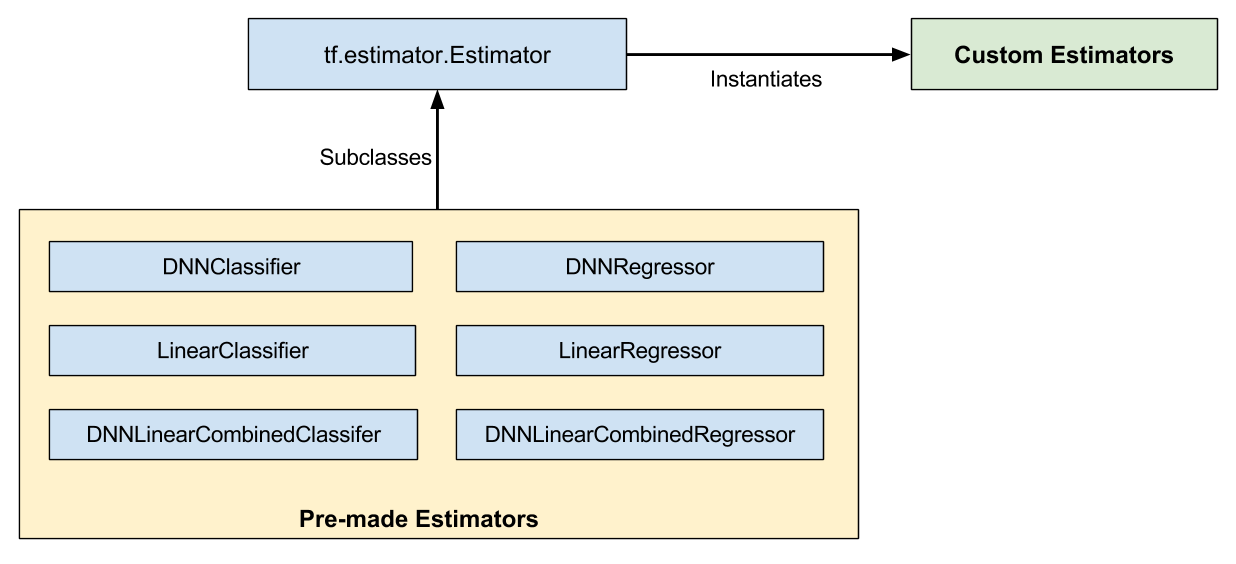• 预制 Estimator，已经有人为您编写了函数模型。
• 定制化 Estimator，您必须自己写函数模型。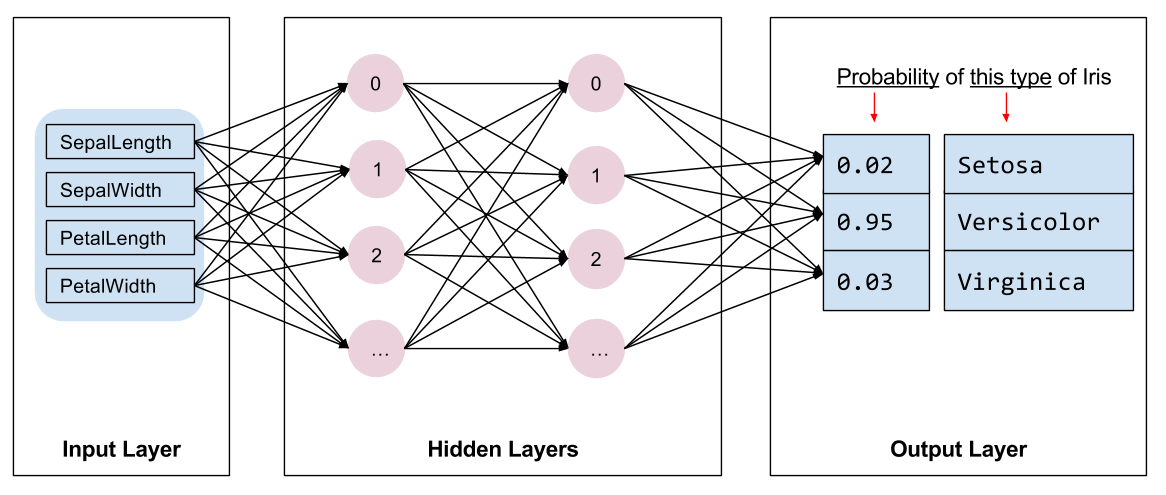## 写一个输入函数

``````def train_input_fn(features, labels, batch_size):
"""An input function for training"""
# 将输入转换为数据集。
dataset = tf.data.Dataset.from_tensor_slices((dict(features), labels))

# 随机播放，重复和批处理示例。
dataset = dataset.shuffle(1000).repeat().batch(batch_size)

# 返回管道读取的结束端。
return dataset.make_one_shot_iterator().get_next()
``````

## 创建功能列

``````# 特征列描述如何使用输入。
my_feature_columns = []
for key in train_x.keys():
my_feature_columns.append(tf.feature_column.numeric_column(key=key))
``````

## 写一个模型函数

``````def my_model_fn(
features, # This is batch_features from input_fn
labels,   # This is batch_labels from input_fn
mode,     # An instance of tf.estimator.ModeKeys
``````

`tf.estimator.DNNClassifier`

``````classifier = tf.estimator.Estimator(
model_fn=my_model,
params={
'feature_columns': my_feature_columns,
# Two hidden layers of 10 nodes each.
'hidden_units': [10, 10],
# The model must choose between 3 classes.
'n_classes': 3,
})
``````

## 定义模型

### 定义输出层

`tf.feature_column.input_layer`

``````    # 使用 `input_layer` 来应用特征列。
net = tf.feature_column.input_layer(features, params['feature_columns'])
``````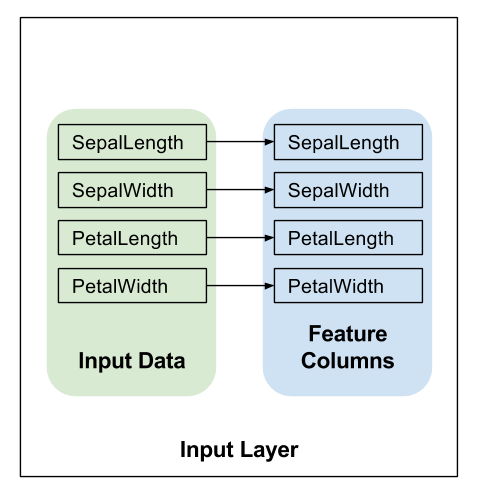### 隐藏层

`tf.layers.dense`

``````    # 根据 'hidden_units' 参数构建隐藏层。
for units in params['hidden_units']:
net = tf.layers.dense(net, units=units, activation=tf.nn.relu)
``````
• `units` 参数定义了给定层中输出神经元的数量。
•  `activation` 参数定义激活函数 — 在本例中为 Relu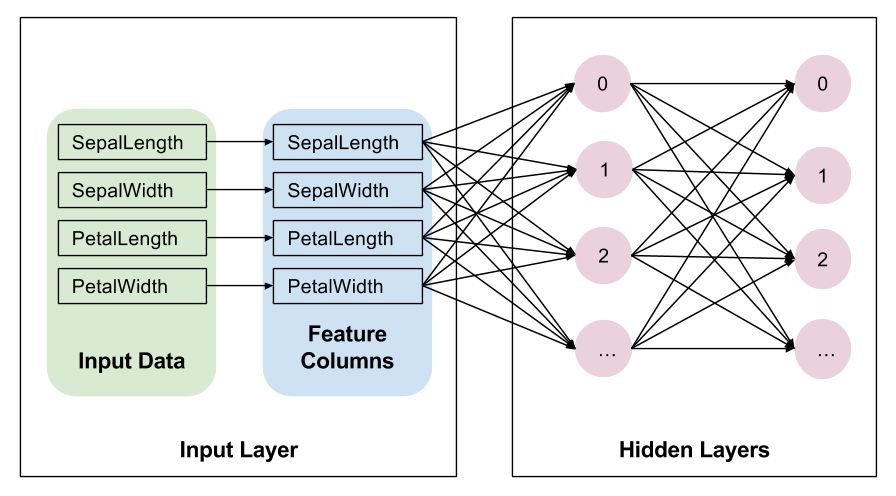`tf.layers.dense`

### 输出层

`tf.layers.dense`

``````    # 计算 logits (每个 class 一个)。
logits = tf.layers.dense(net, params['n_classes'], activation=None)
``````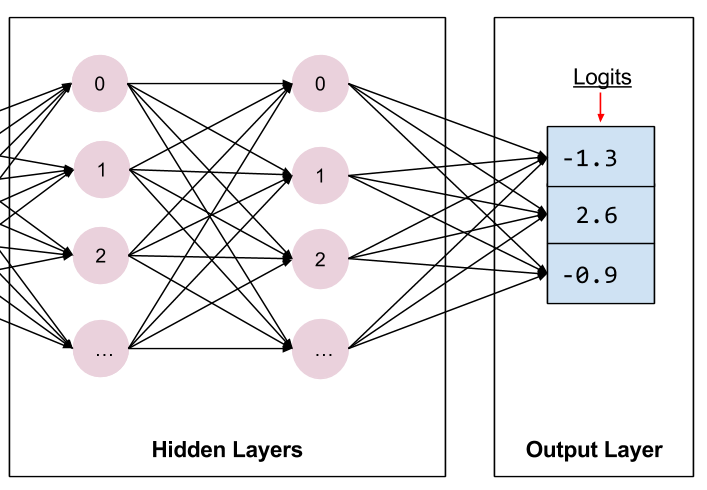`tf.nn.softmax`

## 实现训练、评估、预测 {#modes}

``````def my_model_fn(
features, # This is batch_features from input_fn
labels,   # This is batch_labels from input_fn
mode,     # An instance of tf.estimator.ModeKeys, see below
``````

``````classifier = tf.estimator.Estimator(...)
classifier.train(input_fn=lambda: my_input_fn(FILE_TRAIN, True, 500))
``````

Estimator 框架调用您的函数模型，模式设置为 `ModeKeys.TRAIN`

### 预测

``````#  预测计算
predicted_classes = tf.argmax(logits, 1)
if mode == tf.estimator.ModeKeys.PREDICT:
predictions = {
'class_ids': predicted_classes[:, tf.newaxis],
'probabilities': tf.nn.softmax(logits),
'logits': logits,
}
return tf.estimator.EstimatorSpec(mode, predictions=predictions)
``````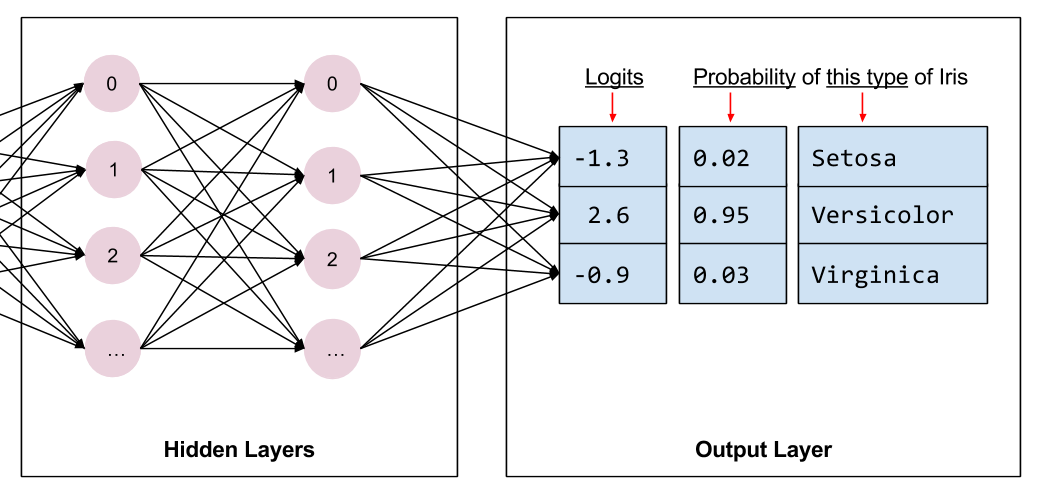`predictions` 包含以下三个键值对：

•   `class_ids` 保存表示模型类 的 id (0, 1, 或者 2)，这个例子中最有可能出现的物种的预测。
•   `probabilities` 保存三个概率 (在本例中 0.02、0.95 和 0.03)。
•   `logit` 保存原始 logit 值 (在本例中 -1.3、2.6 和 -0.9)。

`tf.estimator.Estimator.predict`

### 计算损失

`tf.losses.sparse_softmax_cross_entropy`

``````# 损失计算。
loss = tf.losses.sparse_softmax_cross_entropy(labels=labels, logits=logits)
``````

### 评估

`tf.metrics.accuracy`

``````#  metrics 指标计算。
accuracy = tf.metrics.accuracy(labels=labels,
predictions=predicted_classes,
name='acc_op')
``````

`tf.estimator.EstimatorSpec`

• `loss`，这是模型损失。
• `eval_metric_ops`，这是一个可选的度量字典。

``````metrics = {'accuracy': accuracy}
tf.summary.scalar('accuracy', accuracy)

if mode == tf.estimator.ModeKeys.EVAL:
return tf.estimator.EstimatorSpec(
mode, loss=loss, eval_metric_ops=metrics)
``````

`tf.summary.scalar`

### 训练

`tf.tra.AdagradOptimizer`

``````optimizer = tf.train.AdagradOptimizer(learning_rate=0.1)
``````

`tf.train.Optimizer.minimize`

`tf.train.get_global_step`

``````train_op = optimizer.minimize(loss, global_step=tf.train.get_global_step())
``````

`tf.estimator.EstimatorSpec`

• `loss` 包含损失的函数值。
• `train_op` 执行一个训练步骤。

``````return tf.estimator.EstimatorSpec(mode, loss=loss, train_op=train_op)
``````

## 定制化 Estimator

``````    # 用两个 10 单元建立 2 个隐藏层的 DNN。
classifier = tf.estimator.Estimator(
model_fn=my_model,
params={
'feature_columns': my_feature_columns,
# Two hidden layers of 10 nodes each.
'hidden_units': [10, 10],
# The model must choose between 3 classes.
'n_classes': 3,
})
``````

``````# 训练模型
classifier.train(
input_fn=lambda:iris_data.train_input_fn(train_x, train_y, args.batch_size),
steps=args.train_steps)
``````

## TensorBoard

``````# 将 PATH 替换为以 model_dir 形式传递的实际路径
tensorboard --logdir=PATH
``````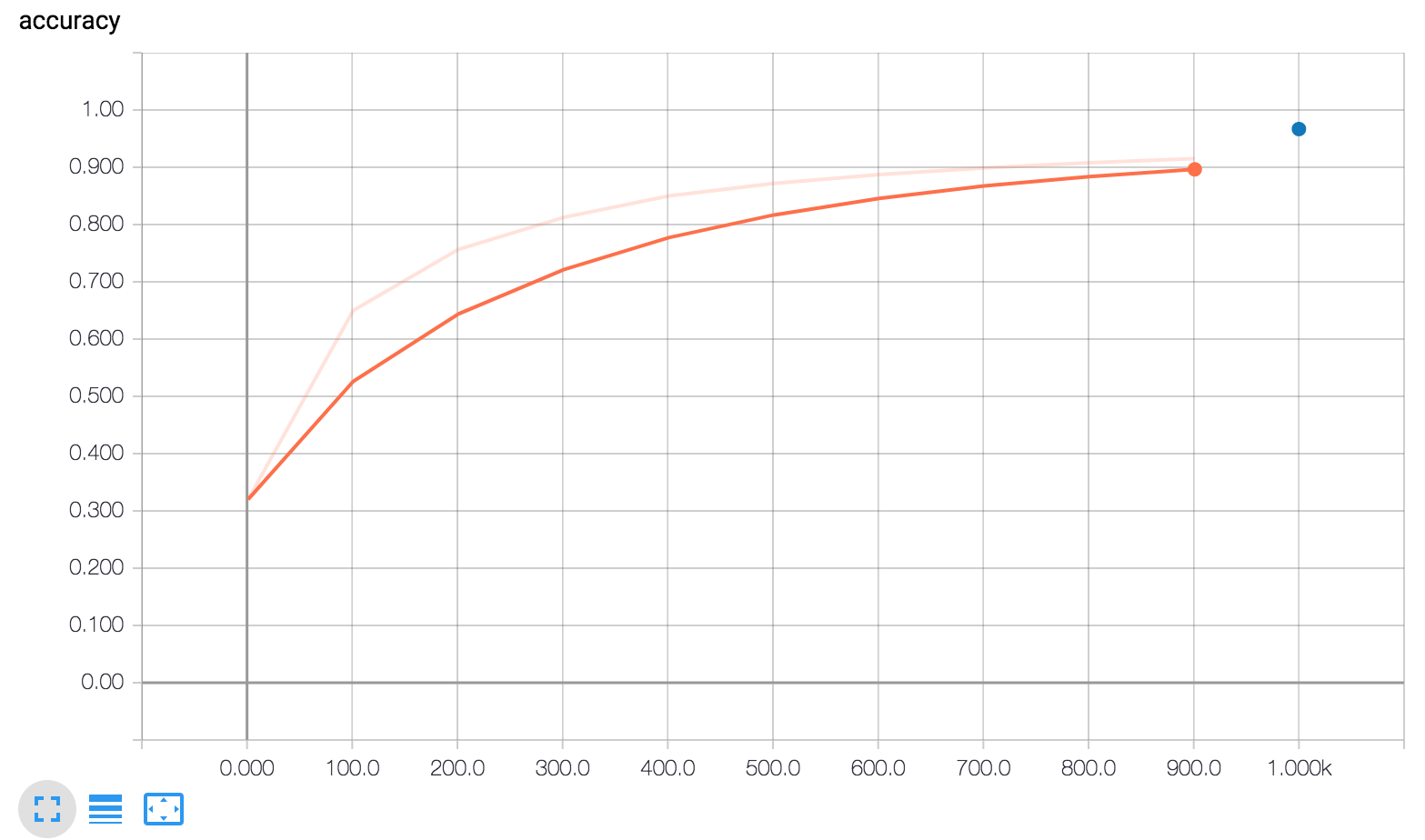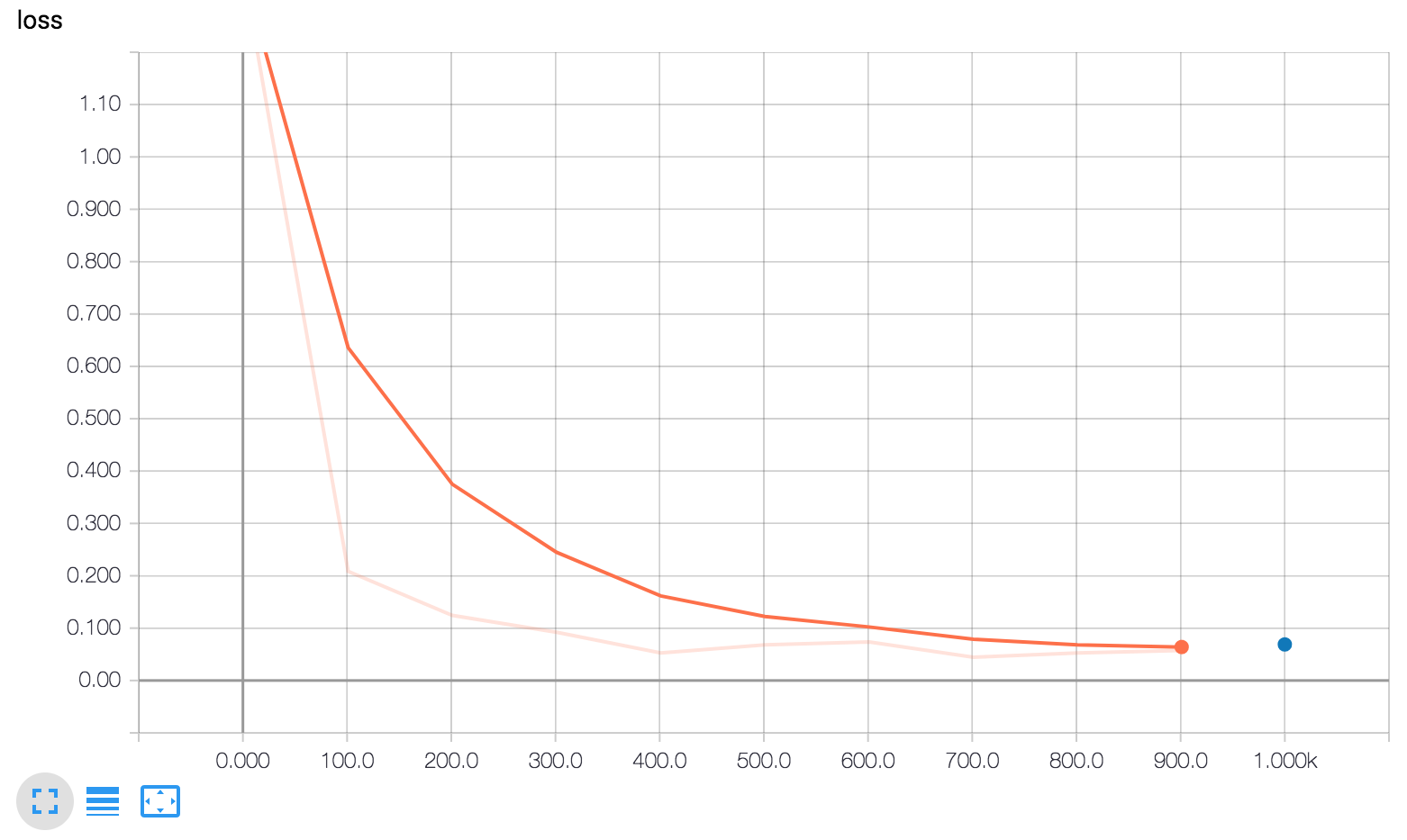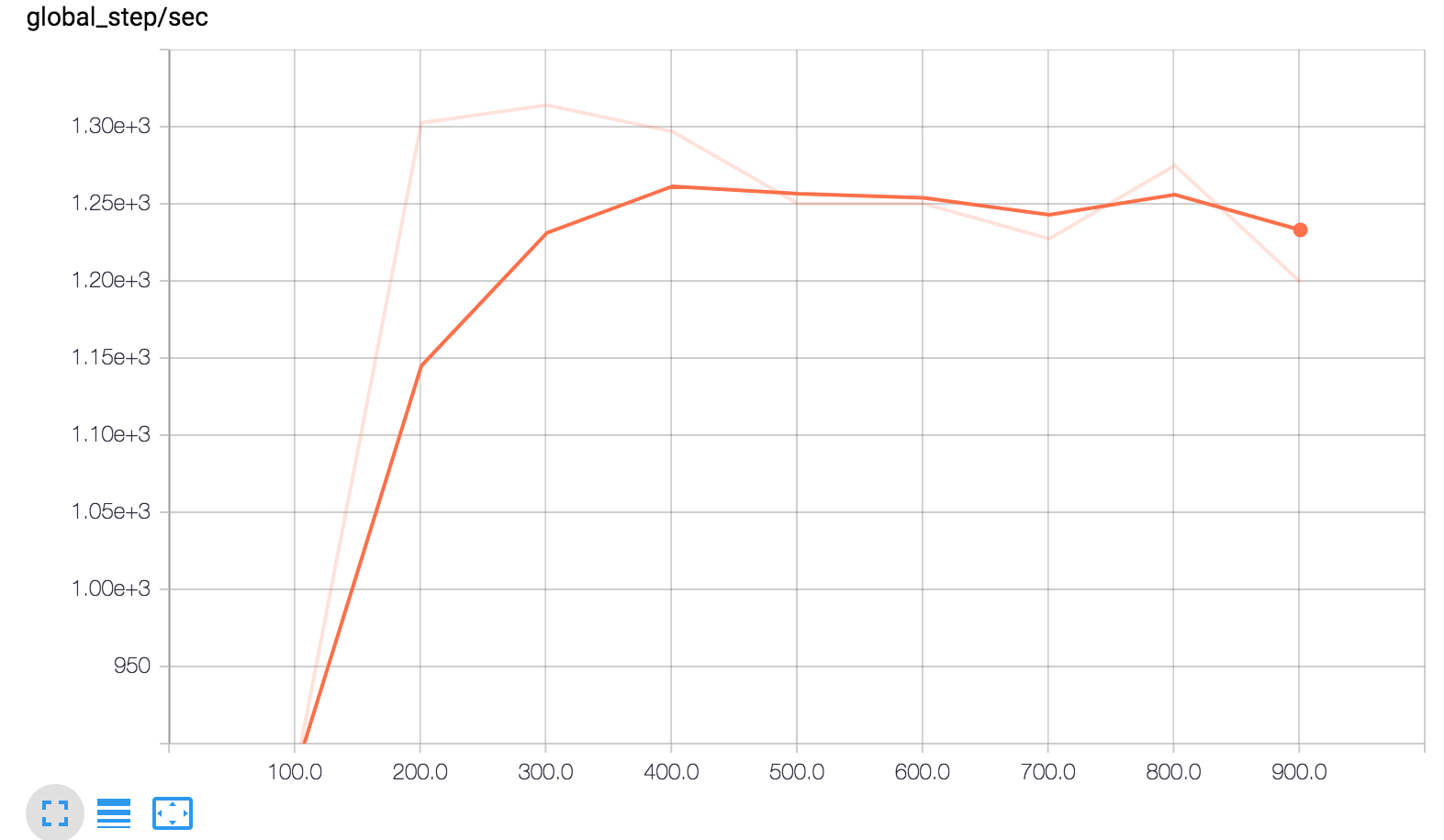TensorBoard 显示三个图形。

• global_step/sec: 显示多少批的性能指标（更新），我们每秒处理的作为训练模型)。

• 损失：损失报告。

• 准确性：准确性由以下两行记录：

• `eval_metric_ops={'my_accuracy': accuracy}`，在评估期间
• `tf.summary.scalar('accuracy', accuracy)`，在训练期间

• 橙色线代表训练。
• 蓝色点代表评估。